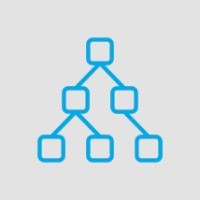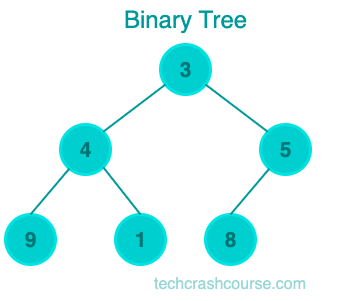# Binary Tree Data Structure# Binary Tree

A binary tree is a tree data structure in which each node can have at most two children, referred to as the left child and the right child. Binary trees are used in computer science to store hierarchical data, such as the file system of a computer.## Types of Binary Tree

• Full Binary Tree: A binary tree is called a full binary tree if every node has either zero or two children. In other words, every node in the binary tree is either a leaf node or has two children.

• Complete Binary Tree: A binary tree is called a complete binary tree if all levels of the tree are completely filled, except possibly the last level, which should be filled from left to right.

• Perfect Binary Tree: A binary tree is called a perfect binary tree if all its levels are completely filled. In other words, a perfect binary tree has 2h - 1 nodes, where h is the height of the tree.

• Balanced Binary Tree: A binary tree is called a balanced binary tree if the height of the left and right subtrees of every node differ by at most one.

• Degenerate (or pathological) Binary Tree: A binary tree is called a degenerate or pathological binary tree if every internal node has only one child, either left or right.

## Uses of Binary Tree

Binary trees are widely used in computer science and programming. Here are some of the common applications of binary trees:
• Search Trees: Binary trees are commonly used as search trees to implement fast searching algorithms. The most common example is the binary search tree, which uses the properties of a binary tree to perform fast searches.
• Expression Trees: Binary trees are used to represent arithmetic expressions, where the leaves represent operands and the internal nodes represent operators. Expression trees are used to evaluate the expression and to convert it into a different form.
• Huffman Coding: Binary trees are used in data compression techniques like Huffman coding, where the binary tree is used to represent a frequency table of characters.
• Network Routing: Binary trees are used to implement network routing algorithms, where the binary tree is used to represent the hierarchical structure of the network.
• Game Trees: Binary trees are used to represent game trees, where the nodes represent the game state and the edges represent the possible moves.
These are just a few of the many applications of binary trees. Understanding binary trees and their properties is essential for many programming tasks and can help in creating efficient algorithms.

## Binary Tree Representation

We can define a binary tree using a struct in C. The struct contains a pointer to the left child and a pointer to the right child. If a node has no children, its left and right child pointers are set to NULL.

```struct Node {
int data;
struct Node* left;
struct Node* right;
};
```
In the above code, we have defined a struct Node that contains an integer data and two pointers to the left and right child nodes. To create a binary tree, we need to write a function to create a new node. The function takes an integer value as a parameter and returns a pointer to the new node.
```struct Node* createNode(int data) {
struct Node* newNode = (struct Node*) malloc(sizeof(struct Node));
newNode->data = data;
newNode->left = NULL;
newNode->right = NULL;
return newNode;
}
```
In the above code, we have defined a function createNode() that creates a new node with the given data value and initializes the left and right child pointers to NULL.

Now, let's create a binary tree using the createNode() function. We will create a binary tree with the following values: 1, 2, 3, 4, 5, 6, and 7.
```struct Node* createBinaryTree() {
struct Node* root = createNode(1);
root->left = createNode(2);
root->right = createNode(3);
root->left->left = createNode(4);
root->left->right = createNode(5);
root->right->left = createNode(6);
root->right->right = createNode(7);
return root;
}
```
In the above code, we have defined a function createBinaryTree() that creates a binary tree with the values 1, 2, 3, 4, 5, 6, and 7. The root node is assigned the value 1, and we have added the left and right children with values 2 and 3, respectively. We have added more nodes to the left and right children of the root node.

## Balanced and Unbalanced Binary Trees

Balanced Binary Trees Unbalanced Binary Trees
A binary tree is considered balanced if the height of the left and right subtrees of any node differs by at most one. A balanced binary tree is essential for achieving optimal time complexity in operations such as insertion, deletion, and searching. An unbalanced binary tree is a tree in which the height of the left and right subtrees of any node can differ by more than one. In an unbalanced binary tree, some subtrees may be deeper than others, and this can cause poor time complexity in operations such as insertion, deletion, and searching.
Balanced binary trees have the advantage of achieving optimal time complexity for common binary tree operations. They also have a more even distribution of nodes, which makes them easier to search and traverse. Unbalanced binary trees, on the other hand, can have poor time complexity for operations such as insertion, deletion, and searching, especially when the tree is highly unbalanced.

## Common Binary Tree Operations

Here are the common operations that can be performed on a binary tree and their time complexity.
• Insertion: Inserting a new node into a binary tree involves finding the appropriate location for the new node and adding it as a leaf node. The time complexity of inserting a node into a binary tree is O(log n) in a balanced tree and O(n) in an unbalanced tree.

• Deletion: Deleting a node from a binary tree involves finding the node to be deleted and then reorganizing the tree to maintain its structure. The time complexity of deleting a node from a binary tree is O(log n) in a balanced tree and O(n) in an unbalanced tree.

• Searching: Searching for a node in a binary tree involves traversing the tree to find the node that matches the search key. The time complexity of searching for a node in a binary tree is O(log n) in a balanced tree and O(n) in an unbalanced tree.

• Traversal: Traversing a binary tree involves visiting each node in the tree in a specific order. There are three common traversal methods: inorder, preorder, and postorder. The time complexity of traversing a binary tree is O(n), where n is the number of nodes in the tree.

• Finding the Height: The height of a binary tree is the maximum number of edges from the root to a leaf node. Finding the height of a binary tree involves traversing the entire tree. The time complexity of finding the height of a binary tree is O(n), where n is the number of nodes in the tree.

• Counting Nodes: Counting the number of nodes in a binary tree involves traversing the entire tree and incrementing a counter for each node. The time complexity of counting the number of nodes in a binary tree is O(n), where n is the number of nodes in the tree.

## Binary Tree Implementation Program

```#include <stdio.h>
#include <stdlib.h>

// Definition of a binary tree node
struct Node {
int data;
struct Node* left;
struct Node* right;
};

// Function to create a new node
struct Node* createNode(int data) {
struct Node* newNode = (struct Node*) malloc(sizeof(struct Node));
newNode->data = data;
newNode->left = NULL;
newNode->right = NULL;
return newNode;
}

struct Node* createBinaryTree() {
struct Node* root = createNode(1);
root->left = createNode(2);
root->right = createNode(3);
root->left->left = createNode(4);
root->left->right = createNode(5);
root->right->left = createNode(6);
root->right->right = createNode(7);
return root;
}

void inorderTraversal(struct Node* root) {
if (root != NULL) {
inorderTraversal(root->left);
printf("%d ", root->data);
inorderTraversal(root->right);
}
}

void preorderTraversal(struct Node* root) {
if (root != NULL) {
printf("%d ", root->data);
preorderTraversal(root->left);
preorderTraversal(root->right);
}
}

void postorderTraversal(struct Node* root) {
if (root != NULL) {
postorderTraversal(root->left);
postorderTraversal(root->right);
printf("%d ", root->data);
}
}

// Main function
int main() {
struct Node* root = createBinaryTree();

printf("Inorder traversal: ");
inorderTraversal(root);
printf("\n");

printf("Preorder traversal: ");
preorderTraversal(root);
printf("\n");

printf("Postorder traversal: ");
postorderTraversal(root);

return 0;
}
```
Output
```Inorder traversal: 4 2 5 1 6 3 7
Preorder traversal: 1 2 4 5 3 6 7
Postorder traversal: 4 5 2 6 7 3 1
```

In the above code, we have defined the main function that calls the createBinaryTree() function to create a binary tree with values 1, 2, 3, 4, 5, 6, and 7. We then call the three traversal functions to print the nodes of the binary tree in the inorder, preorder, and postorder traversal.